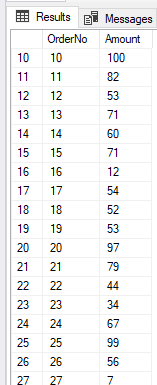﻿ Calculate Sum of Top N Rows using SQL SUM function with Over and Rows ClauseSQL Server and T-SQL Development Tutorials Development resources, articles, tutorials, code samples, tools and downloads for ASP.Net, SQL Server, Reporting Services, T-SQL, Windows, AWS, SAP HANA and ABAP

# SQL SUM function to Calculate Sum of Top N Rows

This SQL tutorial shows how SQL Sum() function is used with OVER clause and ROWS clause to calculate sum of a table column for a number of data rows like TOP 10 rows, etc.

For SQL database developers SUM() aggregation function is one of the first SQL functions learnt during beginning level. Of course when SQL programmers improve their knowledge and know-how on database development, I expect they follow enhancements to aggregate functions like OVER clause and ROWS clausefor example.

Let's assume you have a SQL Server database table with a numeric field which you want to calculate sum its sum.
The simplest method is as follows

Of course you can add filtering criteria using WHERE clause to limit the rows that participate in SUM calculation.
Another example of calculating sum is to calculate the sum of a certain field for top 10 rows.

Let's create a sample database table which contains a numeric column titled Amount.
I'll populate the Amount column with random integer values which I use R script to generate random numbers on SQL Server.

Here is the SQL table DDL script

And following SQL script is used to populate table with random integer values between 1 and 100

Here is the sample table contentsTo calculate the sum of Amount fields for top 10 rows in sample database table, following SQL Select statement can be used

Of course, it is always safer to use an ORDER BY clause with TOP clause in a SELECT command.
Otherwise, SQL developer cannot be sure about the order of the return set
Of course, database programmers can also modify the above code into a SQL CTE expression as follows

Both above SQL codes will produce the same results. And these two SQL Select statements are the standard solutions for a requirement to calculate sum of top N row columns in SQLI will suggest an aother approach for SQL database developers to find the sum of top N rows using SUM() aggregate function with Over Clause using the Rows clause as follows

I experienced huge performance improvements with new solution (Sum aggregate function with Over and Rows clauses) when compared to classic SQL SUM solution especially when database tables with NonClustered indexAs last note, it is always better to try new functions and enhancements to replace old solutions in SQL Server for database programmers if especially there is a requirement for performance improvements.

SQL Server